Next: Estimating the number of Up: Converting Al and Be Previous: Converting the Be/Be ratio

# Uncertainty in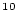Be concentration

The uncertainty in theBe concentration in the quartz sample must take into account three sources of uncertainty: the uncertainty in the isotope ratio measurement (which we will call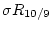), the uncertainty in the number of atoms in the process blanks (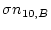), and the uncertainty in the mass of Be added as carrier (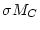). The isotope ratio uncertaintyis supplied by the AMS lab. The blank uncertaintyis discussed below. The uncertainty in the Be carrier mass comes from the fact that the Be carrier is added in solution, and the Be concentration in the solution has some uncertainty. The uncertainty in the Be concentration in typical commercial Be solutions is about 1%, so the uncertainty in the Be carrier mass is typically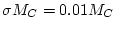.

Using standard error-propagation methods, the formula for the uncertainty in theBe concentration in quartz, that is,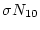, is: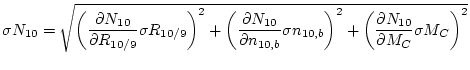(7)

where: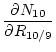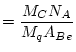(8)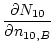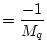(9)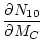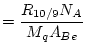(10)Next: Estimating the number of Up: Converting Al and Be Previous: Converting the Be/Be ratio
2006-05-08